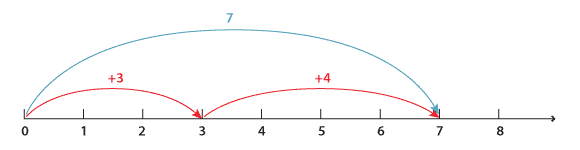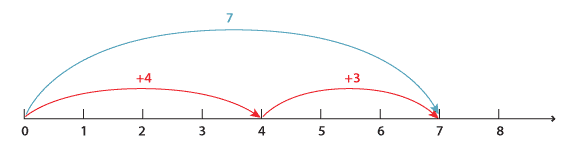Addition satisfies various properties that make calculations easier. The most commonly known law is the commutative law that says, for example, that

3 + 4 = 4 + 3.

It is a mistake to take commutativity for granted or think of it as a trivial observation. Note that subtraction is not commutative (3 − 4 ≠ 4 − 3). In particular, we observe a geometric difference between 3 + 4 and 4 + 3 on the following number lines even though the arithmetic outcome is the same.

3 + 4 corresponds toDetailed description

4 + 3 corresponds toDetailed description